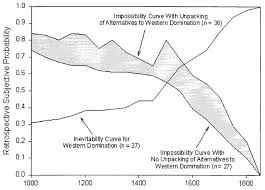## How to Calculate and Solve for Impossibility | ProbabilityThe image above represents impossibility.

To compute for impossibility, one essential parameter is needed and this parameter is Total Number of All Possible Outcomes (N).

The formula for calculating impossibility:

P(A) = x N

Where;

P(A) = Imposssibility
N = Total Number of All Possible Outcomes

Let’s solve an example;
Find the impossibility when the total number of all possible outcomes is 12.

This implies that;

N = Total Number of All Possible Outcomes = 12

P(A) = x N
P(A) = 0 12
P(A) = 0

Therefore, P(impossibility) is 0.